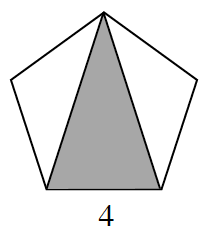### Home > CCG > Chapter 12 > Lesson 12.2.3 > Problem12-85

12-85.

If $\Delta ABC$ is equilateral, and if $A(3, 2)$ and $B(7, 2)$, find all possible coordinates of vertex $C$. Justify your answer. 12-85 HW eTool (Desmos).

There are two possible locations for vertex $C$.Use the eTool below to visualize the problem.
Click the link at right for the full version of the eTool: CCG 12-85 HW eTool